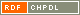# Show document

Title: Reachability relations in digraphs Seifter, Norbert (Author)Zgrablić, Boris (Author)Malnič, Aleksander (Author)Šparl, Primož (Author)Marušič, Dragan (Author)http://dx.doi.org/10.1016/j.ejc.2007.11.003 English Not categorized 1.01 - Original Scientific Article IAM - Andrej Marušič Institute In this paper we study reachability relations on vertices of digraphs, informally defined as follows. First, the weight of a walk is equal to the number of edges traversed in the direction coinciding with their orientation, minus the number of edges traversed in the direction opposite to their orientation. Then, a vertex ▫$u$▫ is ▫$R_k^+$▫-related to a vertex ▫$v$▫ if there exists a 0-weighted walk from ▫$u$▫ to ▫$v$▫ whose every subwalk starting at u has weight in the interval ▫$[0,k]$▫. Similarly, a vertex ▫$u$▫ is ▫$R_k^-$▫-related to a vertex ▫$v$▫ if there exists a 0-weighted walk from ▫$u$▫ to ▫$v$▫ whose every subwalk starting at ▫$u$▫ has weight in the interval ▫$[-k,0]$▫. For all positive integers ▫$k$▫, the relations ▫$R_k^+$▫ and ▫$R_k^-$▫ are equivalence relations on the vertex set of a given digraph. We prove that, for transitive digraphs, properties of these relations are closely related to other properties such as having property ▫$\mathbb{Z}$▫, the number of ends, growth conditions, and vertex degree. graph theory, digraph, reachability relations, end of a graph, property ▫$\mathbb{Z}$▫, growth 2008 str. 1566-1581 Vol. 29, no. 7 0195-6698 519.17 201750910.1016/j.ejc.2007.11.003894 83Document is not linked to any category.

Average score: (0 votes) Voting is allowed only to logged in users.Hover the mouse pointer over a document title to show the abstract or click on the title to get all document metadata.

## Secondary language

Language: English teorija grafov, usmerjeni grafi, rast